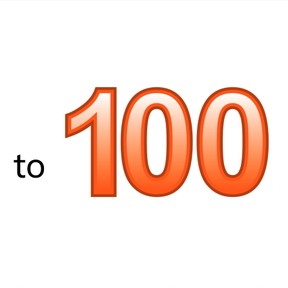Know numbers to 100

# Know numbers to 100

Know numbers to 1008,000 schools use Gynzy92,000 teachers use Gynzy1,600,000 students use Gynzy

## General

Students learn to recognize numbers 21 to 100 from their spoken form and can write down the numbers. Students can say the numbers, write the numbers, and write the numbers in words.

1.NBT.A.1

## Relevance

Discuss with students that numbers are all around us and it is important to recognize them. An example is if you want to know the maximum speed to make sure you don't drive too fast.

## Introduction

Students practice recognizing numbers to 50. Point at numbers in random order and ask students to say them aloud.

## Development

Point at the tens to 100 and say them aloud. Ask students to repeat the numbers after you. Next practice a selection of numbers to 100. Point out that when saying the number, you always start with the ten-fold (twenty-, thirty- forty- etc). Explain how to write the numbers using 79 and 97 as examples. They can be mixed up. Ask students to practice writing numbers. Can they point out the correct answer? Check answers by dragging the boxes. Next practice by saying a number at random and asking students to circle the spoken number.

Check that students have understood by asking the following questions:
Where might you see or find these numbers?
Can you give an example? (speedometer, products, scale)

## Guided Practice

Students first practice by linking a visual number to a spoken number. Next by linking a spoken number to a visual number and finally by linking a written number as numeral.

## Closing

Discuss with students why it is useful to know numbers to 100. Numbers are all around us. An example is if you want to be sure you don't drive faster than the speed limit. Check that students understand by dragging the written numbers to the correct numerals.

## Teaching Tip

Students who have difficulty can be asked to practice saying and writing numbers to 50. Students who are looking for a challenge can be asked to practice saying and writing numbers to 1000.

### The online teaching platform for interactive whiteboards and displays in schools

• Save time building lessons

• Manage the classroom more efficiently

• Increase student engagement